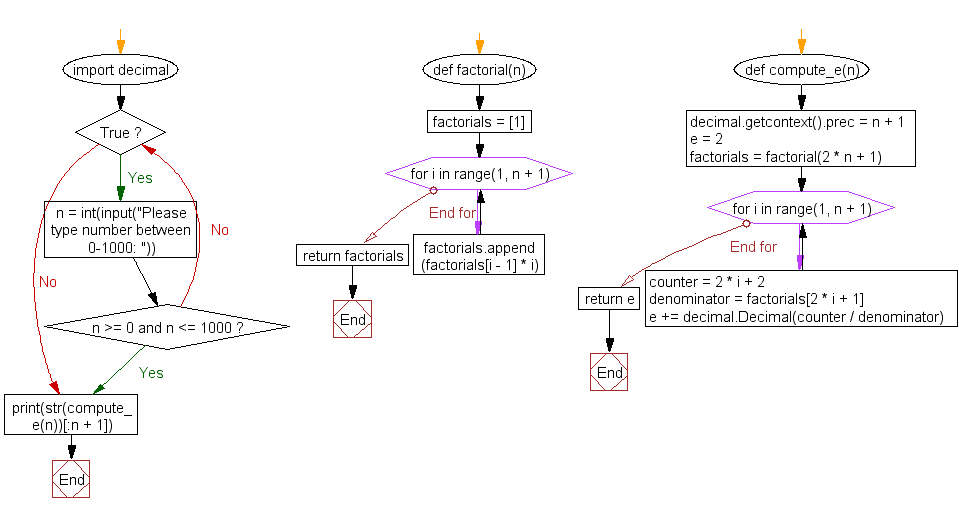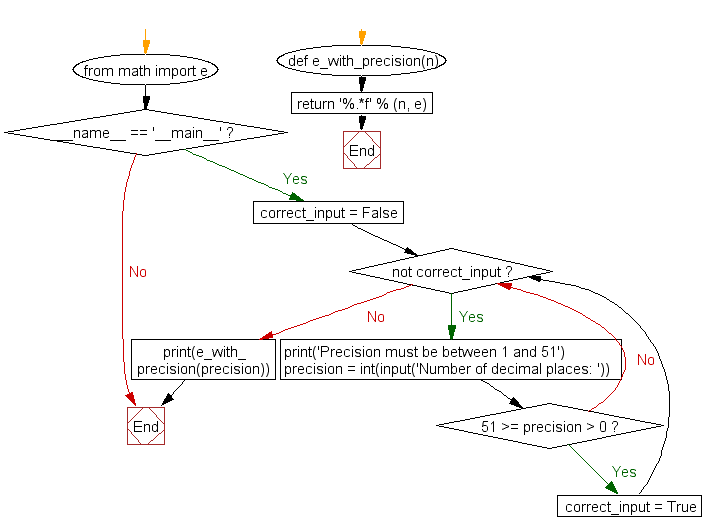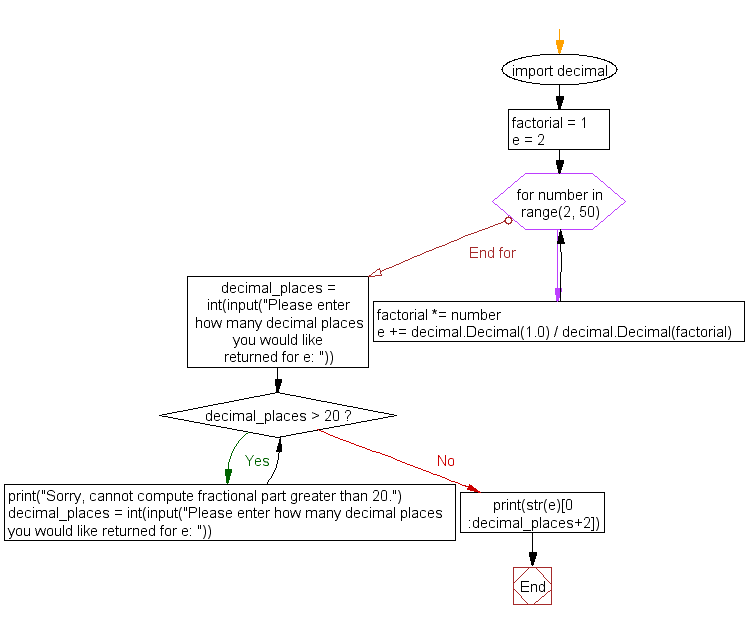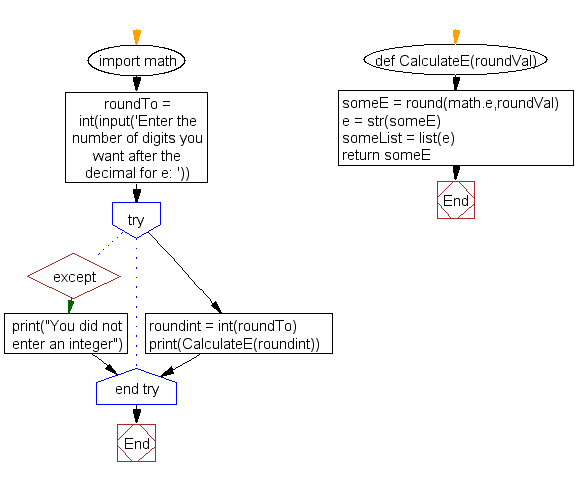﻿ Python Projects: Compute the value of e to n number of decimal places - w3resource

# Python Projects: Compute the value of e to n number of decimal places

## Python Project-2 with Solution

Create a Python project to get the value of e to n number of decimal places.
Note: Input a number and the program will generate e to the 'nth digit.

Sample Solution -1 :

Python Code:

``````# https://github.com/microice333/Python-projects/blob/master/n_digit_e.py
# find e to nth digit by brothers' formulae: http://www.intmath.com/exponential-logarithmic-functions/calculating-e.php
import decimal

def factorial(n):
factorials = 
for i in range(1, n + 1):
factorials.append(factorials[i - 1] * i)
return factorials

def compute_e(n):
decimal.getcontext().prec = n + 1
e = 2
factorials = factorial(2 * n + 1)
for i in range(1, n + 1):
counter = 2 * i + 2
denominator = factorials[2 * i + 1]
e += decimal.Decimal(counter / denominator)
return e

while True:
n = int(input("Please type number between 0-1000: "))
if n >= 0 and n <= 1000:
break

print(str(compute_e(n))[:n + 1])
```
```

Sample Output:

```Please type number between 0-1000: 8
2.7182818
```

Flowchart:Sample Solution -2 :

Python Code:

``````""""Find e to the Nth Digit
Enter a number and have the program generate e up to that many decimal places.
Keep a limit to how far the program will go"""

from math import e

def e_with_precision(n):
"""Return euler's number to the n-th decimal place
:param n: number of decimal places to return
:type n: int
:return: euler's number with n decimal places
:rtype: str
"""
return '%.*f' % (n, e)

if __name__ == '__main__':
# there is no do while loop in python, so we need to improvise
correct_input = False
while not correct_input:
# ask until you get correct input
print('Precision must be between 1 and 51')
precision = int(input('Number of decimal places: '))
if 51 >= precision > 0:
correct_input = True
print(e_with_precision(precision))
``````

Sample Output:

```Precision must be between 1 and 51
Number of decimal places: 5
2.71828
```

Flowchart:Sample Solution -3 :

Python Code:

``````"""
Project: Mega Projects List - Find e to the Nth Digit
Author: Mandeep Bhutani
https://github.com/mandeep/Sample-Projects/blob/master/FindE.py
Problem: Just like the previous problem, but with e instead of Pi.
Enter a number and have the program generate e up to that many decimal places.
Keep a limit to how far the program will go.
References: http://mathworld.wolfram.com/e.html; https://docs.python.org/2/library/decimal.html
"""
import decimal  # Use of decimal module due to increased precision compared to float()

factorial = 1  # The factorial is defined as 1 in order to find e from a nested series.

e = 2  # e is defined as 2 in order to begin the sequence of 2.xxxx.

for number in range(2, 50): # The larger the range, the higher degree of accuracy for euler's number.
# The two lines below perform the nested series calculation in order to find e.
factorial *= number
e += decimal.Decimal(1.0) / decimal.Decimal(factorial)

# Now that e has been calculated, the user can input the number of decimal places desired.

decimal_places = int(input("Please enter how many decimal places you would like returned for e: "))
while decimal_places > 20:
print("Sorry, cannot compute fractional part greater than 20.")
decimal_places = int(input("Please enter how many decimal places you would like returned for e: "))
else:
print(str(e)[0:decimal_places+2])     # The '+2' slice accounts for the beginning two characters of '2.'
``````

Sample Output:

```Please enter how many decimal places you would like returned for e: 5
2.71828
```

Flowchart:Sample Solution -4 :

Python Code:

``````#https://github.com/rlingineni/PythonPractice/blob/master/eCalc/eCalculate.py
import math

def CalculateE(roundVal):

someE = round(math.e,roundVal);
e = str(someE)
someList = list(e)
return someE;
roundTo = int(input('Enter the number of digits you want after the decimal for e: '))
try:
roundint = int(roundTo);
print(CalculateE(roundint));
except:
print("You did not enter an integer");

``````

Sample Output:

```Enter the number of digits you want after the decimal for e: 5
2.71828
```

Flowchart:Improve this sample solutions and post your code through Disqus

﻿